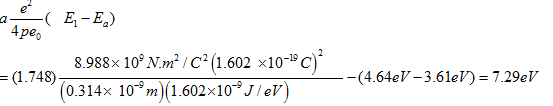# (a) The ionization energy of potassium (K) is 4.34 eV and the electron affinity of chlorine (Cl) is -3.61 eV. The Madelung constant for the KCl structure is 1.748 and the distance between ions of opposite sign is 0.314 nm. On the basis of these data only, compute the cohesive energy of KCl per ion pair.(b) The observed cohesive energy of KCl is 6.42 eV per ion pair. On theassumption that the difference between this figure and that obtained in (a) is due to the exclusion principle repulsion, find the exponent n in the formula Br^-n for the potential energy arising from this source.

Question
20 views

(a) The ionization energy of potassium (K) is 4.34 eV and the electron affinity of chlorine (Cl) is -3.61 eV. The Madelung constant for the KCl structure is 1.748 and the distance between ions of opposite sign is 0.314 nm. On the basis of these data only, compute the cohesive energy of KCl per ion pair.

(b) The observed cohesive energy of KCl is 6.42 eV per ion pair. On the
assumption that the difference between this figure and that obtained in (a) is due to the exclusion principle repulsion, find the exponent n in the formula Br^-n for the potential energy arising from this source.

check_circle

Step 1

a. Cohesive energy will be the negative of the Coulombic energy as given in Numerical, minus the difference between ionization energy of potassium and the electron affinity of chlorine:Hence Cohesive energy for KCL per ion =7.29eV.

...

### Want to see the full answer?

See Solution

#### Want to see this answer and more?

Solutions are written by subject experts who are available 24/7. Questions are typically answered within 1 hour.*

See Solution
*Response times may vary by subject and question.
Tagged in